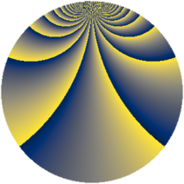# Properties

 Label 1600.2.bnLevel $1600$ Weight $2$ Character orbit 1600.bn Rep. character $\chi_{1600}(149,\cdot)$ Character field $\Q(\zeta_{16})$ Dimension $1136$ Sturm bound $480$

# Related objects

## Defining parameters

 Level: $$N$$ $$=$$ $$1600 = 2^{6} \cdot 5^{2}$$ Weight: $$k$$ $$=$$ $$2$$ Character orbit: $$[\chi]$$ $$=$$ 1600.bn (of order $$16$$ and degree $$8$$) Character conductor: $$\operatorname{cond}(\chi)$$ $$=$$ $$320$$ Character field: $$\Q(\zeta_{16})$$ Sturm bound: $$480$$

## Dimensions

The following table gives the dimensions of various subspaces of $$M_{2}(1600, [\chi])$$.

Total New Old
Modular forms 1968 1168 800
Cusp forms 1872 1136 736
Eisenstein series 96 32 64

## Trace form

 $$1136q + 16q^{4} - 16q^{6} + 16q^{9} + O(q^{10})$$ $$1136q + 16q^{4} - 16q^{6} + 16q^{9} - 16q^{11} + 16q^{14} - 16q^{16} + 16q^{19} - 16q^{21} + 16q^{24} + 64q^{26} + 16q^{29} + 16q^{34} + 144q^{36} + 16q^{39} - 16q^{41} + 16q^{44} - 16q^{46} + 16q^{49} - 80q^{51} - 112q^{54} - 64q^{56} + 144q^{59} - 16q^{61} - 176q^{64} + 208q^{66} + 16q^{69} - 144q^{71} + 64q^{74} + 112q^{76} + 80q^{79} - 16q^{81} + 240q^{84} - 16q^{86} + 16q^{89} - 16q^{91} - 112q^{94} - 16q^{96} - 32q^{99} + O(q^{100})$$

## Decomposition of $$S_{2}^{\mathrm{new}}(1600, [\chi])$$ into newform subspaces

The newforms in this space have not yet been added to the LMFDB.

## Decomposition of $$S_{2}^{\mathrm{old}}(1600, [\chi])$$ into lower level spaces

$$S_{2}^{\mathrm{old}}(1600, [\chi]) \cong$$ $$S_{2}^{\mathrm{new}}(320, [\chi])$$$$^{\oplus 2}$$